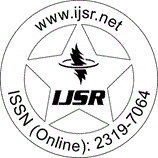International Journal of Science and Research (IJSR)
Call for Papers | Fully Refereed | Open Access | Double Blind Peer Reviewed

Survey Paper | Computer Science & Engineering | India | Volume 6 Issue 1, January 2017

# Efficient Seed and K-Value Selection in K-Means Clustering using Relative Weight and New Distance Metric

Premsagar Dandge | Aruna Gupta 

Abstract: K-mean clustering algorithm is used for clustering the data points which are similar to each other. K-means algorithm is popular due to its simplicity and convergence tendency. The general distance metrics used this algorithm are Euclidian distance, Manhattan distance etc. which are best suited for numeric data like geometric coordinates. These distance metrics does not given full proof results for categorical data. We will be using a new distance metric for calculating the similarity between the categorical data points. The new distance metric uses dynamic attribute weight and frequency probability to differentiate the data points. This ensures the use of categorical properties of the attributes considered while clustering. The k-mean algorithm needs the information about number of clusters present in the dataset in advance before proceeding for cluster analysis. We will be using a different technique for finding out the number of clusters which is based on the data density distribution. Also the initial cluster seeds are selected in a random fashion which may lead to more iteration required for convergent solution. In proposed method, seeds are selected considering the density distribution which ensures the even distribution of initial seed selection. This will reduce the overall iteration required for convergent solution.

Keywords: k-means clustering, categorical data, dynamic attribute weight, frequency probability, data density

Edition: Volume 6 Issue 1, January 2017,

Pages: 2084 - 2087